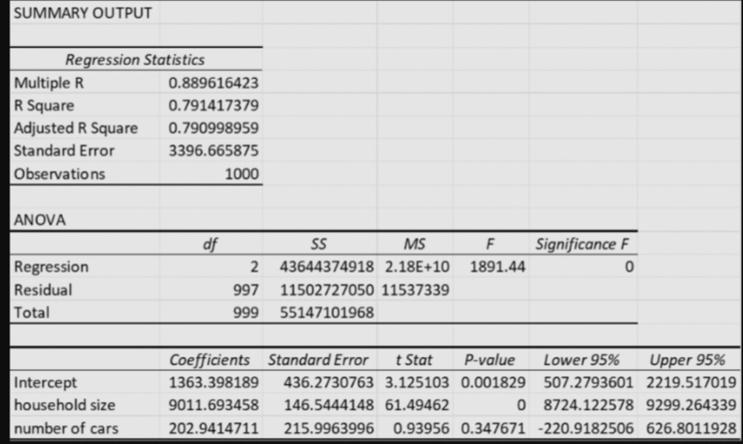Home / Expert Answers / Statistics and Probability / the-output-below-refers-to-the-total-distance-travelled-by-car-by-members-of-a-household-in-a-year-a-pa620

# (Solved): The output below refers to the total distance travelled by car by members of a household in a year a ...

The output below refers to the total distance travelled by car by members of a household in a year as “distance travelled by a household”. Travel distance by household (km) is closely related to household size and number of cars owned or leased.

The following is an extract from the regression output:What does the regression output tell you about the direction of the relationship between the variables? Briefly explain the reasoning behind your answer. (2 marks)

What is the percentage of the variation in household travel distance that is explained by the regression (1 mark)

Using a 5% level of significance, conduct a hypothesis test to determine whether there is evidence that a linear relationship exists between household travel distance and each explanatory variable. Use the p-value approach. (4 marks)

If we decide to run a simple linear regression instead, using only number of cars as the independent variable, would R2 be higher or lower? Provide an explanation by referring to variables and their statistical significance. (3 marks)

SUMMARY OUTPUT \begin{tabular}{lr} \hline \multicolumn{2}{c}{ Regression Statistics } \\ \hline Multiple R & \\ R Square & \\ Adjusted R Square & \\ Standard Error & \\ Observations & 1000 \\ \hline \end{tabular} ANOVA \begin{tabular}{lrrcccr} \hline & & \multicolumn{1}{c}{ SS } & MS & & Significance \\ \hline Regression & 2 & 43644374918 & & & 0 \\ Residual & 997 & 11502727050 & 11537339 & & \\ Total & 999 & 55147101968 & & & \\ \hline \end{tabular} \begin{tabular}{llrrrrr} \hline & Coefficients & Standard Error & \multicolumn{1}{c}{ Stat } & \multicolumn{1}{c}{ P-value } & \multicolumn{1}{c}{ Lower 95\% } & Upper 95\% \\ \hline Intercept & & & & & & \\ household size & & & & 0 & & \\ number of cars & & & & & & \\ \hline \end{tabular}

We have an Answer from Expert